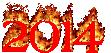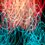# Can you beat this burning 2014 game?What is the smallest positive integer which cannot be formed using the digits 2, 0, 1, 4 in that order? You are allowed to use $+, -, \times, \div$, exponential, factorials, etc. Be creative, and share what you can get. Let's see how high we can go.

To get started, I have:
$1 = -2 - 0 - 1 + 4$
$2 = - 2 - 0 \times 1 + 4$
$3 = 2 \times 0 - 1 + 4$
$4 = 2 \times 0 \times 1 + 4$

For clarity, the only numbers which appear are $2, 0, 1, 4$ in that order. You cannot use a greek symbol to present a number ( e.g. $\pi = 3.14159\ldots$. The square root sign $\sqrt{ \, }$ is borderline, since it actually represents $\sqrt{ \, }$, but we'd allow it. You can't use cube root, unless you have a 3, as in $\sqrt{ \, }$.Note by Chung Kevin
7 years ago

This discussion board is a place to discuss our Daily Challenges and the math and science related to those challenges. Explanations are more than just a solution — they should explain the steps and thinking strategies that you used to obtain the solution. Comments should further the discussion of math and science.

When posting on Brilliant:

• Use the emojis to react to an explanation, whether you're congratulating a job well done , or just really confused .
• Ask specific questions about the challenge or the steps in somebody's explanation. Well-posed questions can add a lot to the discussion, but posting "I don't understand!" doesn't help anyone.
• Try to contribute something new to the discussion, whether it is an extension, generalization or other idea related to the challenge.

MarkdownAppears as
*italics* or _italics_ italics
**bold** or __bold__ bold
- bulleted- list
• bulleted
• list
1. numbered2. list
1. numbered
2. list
Note: you must add a full line of space before and after lists for them to show up correctly
paragraph 1paragraph 2

paragraph 1

paragraph 2

[example link](https://brilliant.org)example link
> This is a quote
This is a quote
    # I indented these lines
# 4 spaces, and now they show
# up as a code block.

print "hello world"
# I indented these lines
# 4 spaces, and now they show
# up as a code block.

print "hello world"
MathAppears as
Remember to wrap math in $$ ... $$ or $ ... $ to ensure proper formatting.
2 \times 3 $2 \times 3$
2^{34} $2^{34}$
a_{i-1} $a_{i-1}$
\frac{2}{3} $\frac{2}{3}$
\sqrt{2} $\sqrt{2}$
\sum_{i=1}^3 $\sum_{i=1}^3$
\sin \theta $\sin \theta$
\boxed{123} $\boxed{123}$

Sort by:

5 = 2+0-1 + 4

6 = 2 + 0 X 1 + 4

7 = 2 + 0 + 1 + 4

8 = (2 + 0 X 1) X 4

I can't get 9, maybe something like 2X4 +1?

- 7 years ago

$9=\sqrt{(2+0+1)^4}$ is allowed?

- 7 years ago

If that isn't allowed, there's always $(-2-0!)\cdot (1-4)$.

- 7 years ago

$10=(2+0)\times(1+4)$

Pero no puedo obtener 11...

- 7 years ago

$11=(2+0!)!+1+4$

- 7 years ago

$12=(2+0+1)\times 4$

$13=-2^0+14$

$14=2\times 0+14$

$15=2^0+14$

$16=2+0+14$

$17=2+0!+14$

$18=2+(0!+1)^4$

$19=20-1^4$

$20=(2+0!)!+14$

$21=-2-0-1+4!$

$22=-2+0\times 1+4!$

$23=2\times 0-1+4!$

$24=2\times 0\times 1+4!$

$25=2\times 0+1+4!$

$26=2+0\times 1+4!$

$27=2+0+1+4!$

$28=2+0!+1+4!$

$29=(2+0!)!-1+4!$

$30=(2+0!)!\times 1+4!$

$31=(2+0!)!+1+4!$

$32=2((0!+1)^4)$

$33=\phi((2+0!+1)!)+4!$

$34=20+14$

Is combining digits allowed? How about totient function?

- 7 years ago

$35 = \left \lfloor \sqrt{ \left ( (2+0!)! + 1 \right )! } \right \rfloor \div \sqrt {4}$

$36 = \sqrt {\left ( 2+0! \right )!^{(1 \times 4)} }$

$37 = \left \lfloor \sqrt{ \phi ( ( ((2+0!)!)!) } \right \rfloor + (1 \times 4!)$

$38 = \left \lceil \sqrt{ \phi ( ( ((2+0!)!)!) } \right \rceil + ( 1 \times 4!)$

$39 = \left \lceil \sqrt{ \phi ( ( ((2+0!)!)!) } \right \rceil + 1 + 4!$

$40 = \sqrt { \phi \left ( \phi \left ( (2+0!)! \right ) \right ) } \times (1 + 4)$

$41 = \left \lceil \sqrt{((2+0!)!)!} \right \rceil + 14$

$42 = \phi \left ( \left \lceil \sqrt{((2+0!)!)!} \right \rceil \right ) + 1 \times 4!$

$43 = \phi \left ( \left \lceil \sqrt{((2+0!)!)!} \right \rceil \right ) + 1 + 4!$

$44 = \left \lfloor \sqrt{2014} \right \rfloor$

$45 = \left \lceil \sqrt{2014} \right \rceil$

$46 = \phi \left ( \left ( \left \lceil \sqrt {\sqrt { \phi ( ((2+0!)!)! )} } \right \rceil \right ) ! - 1 + 4! \right )$

$47 = \left ( \left \lceil \sqrt {\sqrt { \phi ( ((2+0!)!)! )} } \right \rceil \right ) ! - 1 + 4!$

$48 = (2+0!+1)! +4!$

$49 = \left ( \left \lceil \sqrt {\sqrt { \phi ( ((2+0!)!)! )} } \right \rceil \right ) ! + 1 + 4!$

$50 = \left \lfloor 201 \div 4 \right \rfloor$

$51 = \left \lceil 201 \div 4 \right \rceil$

$52 = \left \lfloor \sqrt { \phi \left ( ((2+0!)!)! \right ) } \right \rfloor \times 1 \times 4$

$53 = \phi \left ( \left \lfloor \sqrt { \sqrt { \sqrt{20!}}} \right \rfloor \right ) + 1 - \phi (4!)$

$54 = \phi \left ( \left \lceil \sqrt{ ((2+0!)!)! } \right \rceil \right ) \times 1 \times \left \lfloor \sqrt {\phi (4!) } \right \rfloor$

$55 = \phi ( \phi ( \phi ( ((2+0!)!)!))) - 1 + 4!$

$56 = \left \lceil \sqrt{ \phi ( ((2+0!)!)! ) } \right \rceil \times 1 \times \left \lceil \phi ( \phi (4!)) \right \rceil$

$57 = \phi ( \phi ( \phi ( ((2+0!)!)!))) + 1 + 4!$

$58 = \phi ( \phi ( ((2+0!)!)!)) - \left \lceil \sqrt{ \phi ( ( 1+4)! ) } \right \rceil$

$59 = \phi ( \phi ( ((2+0!)!)!)) - 1 - 4$

$60 = \phi ( \phi ( ((2+0!)!)!)) - ( 1 \times 4)$

$61 = \phi ( \phi ( ((2+0!)!)!)) + 1 - 4$

$62 = \phi ( \phi ( ((2+0!)!)!)) - ( 1 \times \sqrt{4})$

$63 = \phi ( \phi ( ((2+0!)!)!)) + 1 - \sqrt{4}$

$64 = \left ( \phi ( \phi ( ((2+0!)!)!)) \right ) \times (1^4)$

$65 = \phi ( \phi ( ((2+0!)!)!)) + 1 + \sqrt{4}$

$66 = \left ( \phi ( \phi ( ((2+0!)!)!)) \right ) \times 1 + \sqrt{4}$

- 7 years ago

Oh wow. It seems possible that with factorial, totient, floor/ceiling and roots, you can get wriggle around a lot.

What if square roots were not allowed?

Staff - 7 years ago

Oh actually I can construct every positive integer. Take $((((((0+1+4)!)!)!)!)!)!$, or an arbitrarily large number of factorials, then apply the totient function a number of times such that the number is the desired power of 2, then take $\log\ 2$. $n=\log_2 (\phi(\phi(\cdots(\phi(\phi(((\cdots((0+1+4)!)!\cdots)!)!)\cdots)))$ This works since repeatedly applying the totient function eventually gives a power of 2, and the totient of a power of 2 is the previous power of 2.

For example, $50=\log_2(\phi^{501}(((0+1+4)!)!))$ where $\phi^n$ denotes applying the totient function $n$ times. Calculated via Mathematica.

- 7 years ago

Why does totient eventually make a power of 2?

- 7 years ago

First, I'll assume you know the formula for the totient function.

When applying totient to an odd prime, another 2, along with possibly some other odd primes, is generated. With each totient, the power on 2 increases/stays the same unless the number is a power of 2. Eventually, all odd primes are gone, and there is a large power of 2 remaining.

- 7 years ago

That's pretty cool :) Of course, the next question is, what happens if the totient function is not allowed?

- 7 years ago

Well, without the totient function, I can't find a way to make 33.

- 7 years ago

$35=\phi(\phi(\phi((2+0!)!)))-1-4$

$36=\phi(\phi(\phi((2+0!)!)))\times 1-4$

$37=\phi(\phi(\phi((2+0!)!)))+1-4$

$38=\phi(\phi(\phi((2+0!)!)))-1\times\phi(4)$

$39=\phi(\phi(\phi((2+0!)!)))+1-\phi(4)$

$40=\phi(\phi(\phi((2+0!)!)))+1-\phi(\phi(4))$

$41=\phi(\phi(\phi((2+0!)!)))+1\times\phi(\phi(4))$

$42=\phi(\phi(\phi((2+0!)!)))+1\times \phi(4)$

$43=\phi(\phi(\phi((2+0!)!)))+1+\phi(4)$

$44=\phi(\phi(\phi((2+0!)!)))+1\times 4$

$45=\phi(\phi(\phi((2+0!)!)))+1+4$

$46=-2+(0!+1)\times 4!$

$47=-\phi(2)+(0!+1)\times 4!$

$48=(2+0\times 1)\times 4!$

$49=\phi(2)+(0!+1)\times 4!$

$50=2+(0!+1)\times 4!$

$51=(2+0!)\times(1+\phi^9((\phi(4!)!)))$

- 7 years ago

$48=\phi(20)\times\phi(14)$

- 6 years ago

I honestly don't know, been trying hard to continue after $34$ without the use of square root. Turns out it works very handy with floor/ceiling function and factorials. This is because when we combine $2$ and $0$ as such: $((2+0!)!)! = 720$, and apply the functions in different orders, we can get many (possibly infinite) natural numbers.

- 7 years ago

$67 = \phi(\phi((((2+0!)!)!))) - 1 + 4$

$68 = \phi(\phi((((2+0!)!)!))) \times 1 + 4$

$69 = \phi(\phi((((2+0!)!)!))) + 1 + 4$

$70 = \phi(\phi((((2+0!)!)!))) + (1 + |\sqrt{4}|)!$

$71 = \phi(\phi((((2+0!)!)!))) - 1 + \phi(4!)$

$72 = \phi(\phi((((2+0!)!)!))) \times 1 + \phi(4!)$

$73 = \phi(\phi((((2+0!)!)!))) + 1 + \phi(4!)$

$74 = \phi(\phi((((2+0!)!)!))) + \phi(\phi(-1+4!))$

$75 = (2+0!) \times (1+4!)$

$76 = (20-1) \times 4$

64 can also equal $4^{(2+1)} \times 0!$

- 7 years ago

33 is also $\frac{\varphi(201)}{4}$

- 7 years ago

This is probably a better solution since $\phi ((2+0!+1)!) +4! = \phi (24) + 24 = 8 + 24 = 32$ not $33$.

- 7 years ago

True enough I messed up, nice catch!

- 7 years ago

Factorial.....

You're smart lol

- 7 years ago

[(2+0)x4]+1

- 7 years ago

Well, this is in the wrong order.

- 7 years ago

True, we always depend on other functions, disregarding the basic stuffs.

- 7 years ago

(0!+0!+0!+0!+0!+0!)!=720

- 7 years ago

2 raised to 0 +1 raised to 4

- 7 years ago

5 = 2+0-1 + 4

6 = 2 + 0 X 1 + 4

7 = 2 + 0 + 1 + 4

8 = (2 + 0 X 1) X 4

- 7 years ago

maybe every number can be formed

- 7 years ago

$1,638,400,000,000,000,000=20^{14}$

$2,432,902,008,176,640,000^{87,178,291,200} = 20!^{14!}$ is a possibility as well if you can use double digits

Noone told me me anything not being able to:

$2,432,902,008,176,640,000^{87,178,291,200}! = (20!^{14!})!$

- 6 years, 11 months ago

$100=20\times(1+4)$

- 6 years, 1 month ago

Are there any people from 2018 that are commenting on this apart from me?

- 2 years, 2 months ago

9 = [(2+0)x4]+1

- 7 years ago